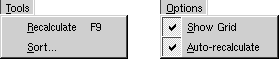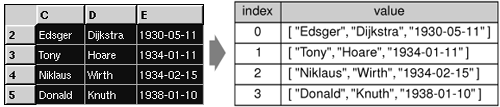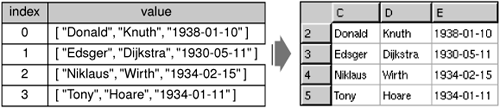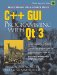We will now implement the slots for the Tools and Options menus.```void Spreadsheet::recalculate()
{
int row;

for (row = 0; row < NumRows; ++row) {
for (int col = 0; col < NumCols; ++col) {
if (cell(row, col))
cell(row, col)->setDirty();
}
}
for (row = 0; row < NumRows; ++row) {
for (int col = 0; col < NumCols; ++col) {
if (cell(row, col))
updateCell(row, col);
}
}
}
```

The recalculate() slot corresponds to Tools|Recalculate. It is also called automatically by Spreadsheet when necessary.

We iterate over all the cells and call setDirty() on every cell to mark each one as requiring recalculation. The next time QTable calls text() on a Cell to obtain the value to show in the spreadsheet, the value will be recalculated.

Then we call updateCell() on all the cells to repaint the whole spreadsheet. The repaint code in QTable then calls text() on each visible cell to obtain the value to display. Because we called setDirty() on every cell, the calls to text() will use a freshly calculated value. The calculation is performed by the Cell class.

```void Spreadsheet::setAutoRecalculate(bool on)
{
autoRecalc = on;
if (autoRecalc)
recalculate();
}
```

The setAutoRecalculate() slot corresponds to Options|Auto-recalculate. If the feature is turned on, we recalculate the whole spreadsheet immediately to make sure that it's up to date. Afterward, recalculate() is called automatically from somethingChanged().

We don't need to implement anything for Options|Show Grid because QTable already provides a setShowGrid(bool) slot. All that remains is Spreadsheet::sort(), which we called from MainWindow::sort():

```void Spreadsheet::sort(const SpreadsheetCompare &compare)
{
vector rows;
QTableSelection sel = selection();
int i;

for (i = 0; i < sel.numRows(); ++i) {
QStringList row;
for (int j = 0; j < sel.numCols(); ++j)
row.push_back(formula(sel.topRow() + i,
sel.leftCol() + j));
rows.push_back(row);
}

stable_sort(rows.begin(), rows.end(), compare);

for (i = 0; i < sel.numRows(); ++i) {
for (int j = 0; j < sel.numCols(); ++j)
setFormula(sel.topRow() + i, sel.leftCol() + j,
rows[i][j]);
}

clearSelection();
somethingChanged();
}
```

Sorting operates on the current selection and reorders the rows according to the sort keys and sort orders stored in the compare object. We represent each row of data with a QStringList and store the selection as a vector of rows. The vector class is a standard C++ class; it is explained in Chapter 11 (Container Classes). For simplicity, we sort by formula rather than by value.

Figure 4.10. Storing the selection as a vector of rowsWe call the standard C++ stable_sort() function on the rows to perform the actual sorting. The stable_sort() function accepts a begin iterator, an end iterator, and a comparison function. The comparison function is a function that takes two arguments (two QStringLists) and that returns true if the first argument is "less than" the second argument, false otherwise. The compare object we pass as the comparison function isn't really a function, but it can be used as one, as we will see shortly.

Figure 4.11. Putting the data back into the table after sortingAfter performing the stable_sort(), we move the data back into the table, clear the selection, and call something Changed().

```class SpreadsheetCompare
{
public:
bool operator()(const QStringList &row1,
const QStringList &row2) const;

enum { NumKeys = 3 };
int keys[NumKeys];
bool ascending[NumKeys];
};
```

The SpreadsheetCompare class is special because it implements a () operator. This allows us to use the class as if it were a function. Such classes are called functors. To understand how functors work, we will start with a simple example:

```class Square
{
public:
int operator()(int x) const { return x * x; }
};
```

The Square class provides one function, operator()(int), that returns the square of its parameter. By naming the function operator()(int) rather than, say, compute(int), we gain the capability of using an object of type Square as if it were a function:

```Square square;
int y = square(5);
```

Now let's see an example involving SpreadsheetCompare:

```QStringList row1, row2;
...
if (compare(row1, row2)) {
// row1 is less than row2
}
```

The compare object can be used just as if it had been a plain compare() function. Additionally, it can access all the sort keys and sort orders, which it stores as member variables.

An alternative to this scheme would have been to store the sort keys and sort orders in global variables and use a plain compare() function. However, communicating through global variables is inelegant and can lead to subtle bugs. Functors are a more powerful idiom for interfacing with template functions such as stable_sort().

Here is the implementation of the function that is used to compare two spreadsheet rows:

```bool SpreadsheetCompare::operator() (const QStringList &row1,
const QStringList &row2) const
{
for (int i = 0; i < NumKeys; ++i) {
int column = keys[i];
if (column != -1) {
if (row1[column] != row2[column]) {
if (ascending[i])
return row1[column] < row2[column];
else
return row1[column] > row2[column];
}
}
}
return false;
}
```

It returns true if the first row is less than the second row; otherwise, it returns false. The standard stable_sort() function uses the result of this function to perform the sort.

The SpreadsheetCompare object's keys and ascending arrays are populated in the MainWindow::sort() function (shown in Chapter 2). Each key holds a column index, or 1 ("None").

We compare the corresponding cell entries in the two rows for each key in order. As soon as we find a difference, we return an appropriate true or false value. If all the comparisons turn out to be equal, we return false. The stable_sort() function uses the order before the sort to resolve tie situations; if row1 preceded row2 originally and neither compares as "less than" the other, row1 will still precede row2 in the result. This is what distinguishes std::stable_sort() from its more famous (but less stable) cousin std::sort().

We have now completed the Spreadsheet class. In the next section, we will review the Cell class. This class is used to hold cell formulas and provides a reimplementation of the text() function that Spreadsheet calls to display the result of calculating a cell's formula.C++ GUI Programming with Qt 3
ISBN: 0131240722
EAN: 2147483647
Year: 2006
Pages: 140